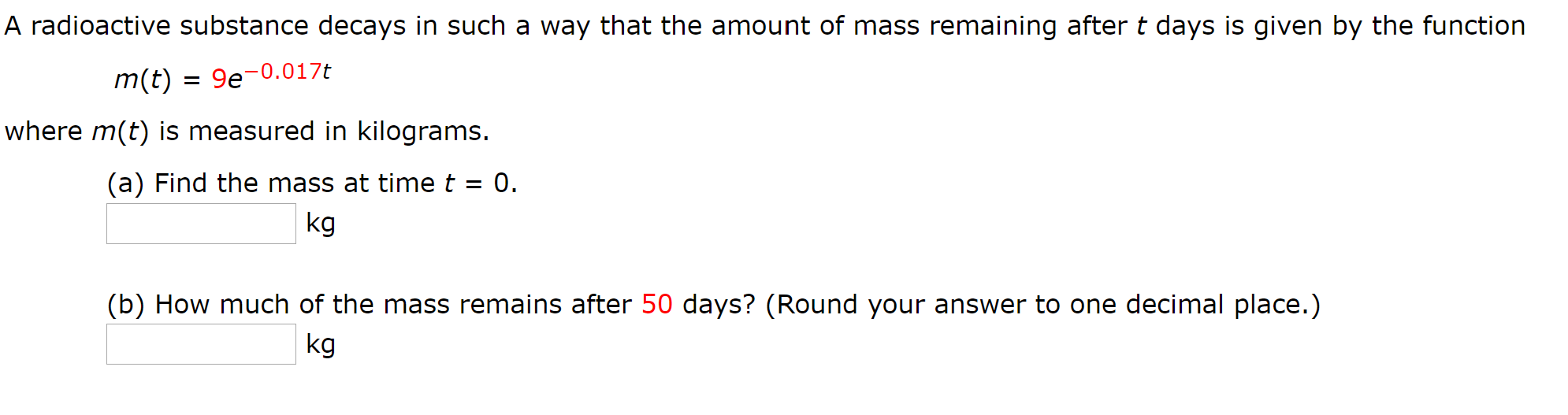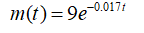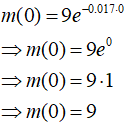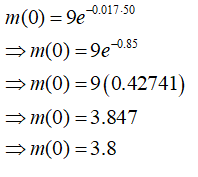# A radioactive substance decays in such a way that the amount of mass remaining after t days is given by the functionm(t)= 9e-0.017twhere m(t) is measured in kilograms.(a) Find the mass at timet = 0.%3Dkg(b) How much of the mass remains after 50 days? (Round your answer to one decimal place.)kg

Question
2 viewshelp_outlineImage TranscriptioncloseA radioactive substance decays in such a way that the amount of mass remaining after t days is given by the function m(t) = 9e-0.017t where m(t) is measured in kilograms. (a) Find the mass at timet = 0. %3D kg (b) How much of the mass remains after 50 days? (Round your answer to one decimal place.) kg fullscreen
check_circle

Step 1

Given:Step 2

Part (a)

Plugging t=0, we get:Mass at time t=0 is 9 kg

Step 3

Part (b)

Plugging t=50, we get:Mass remaining after 50 days is 3.8 kg

...

### Want to see the full answer?

See Solution

#### Want to see this answer and more?

Solutions are written by subject experts who are available 24/7. Questions are typically answered within 1 hour.*

See Solution
*Response times may vary by subject and question.
Tagged in